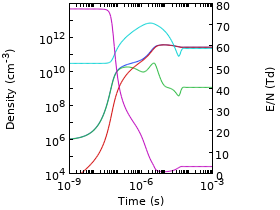Application Gallery

Global Model Coupled with the Two-Term Boltzmann Equation

Application ID: 67411

The electron energy distribution function (EEDF) plays an important role in the overall behavior of discharges. In this example, the formation period of an Argon plasma is studied with special attention paid to the EEDF. The plasma is created within a 4 cm gap by a DC source voltage of 1 kV at 100 mTorr. A global model in the local field approximation is used to describe the temporal evolution of the plasma species. The rate coefficients for electron impact reactions and electron mobility are obtained from suitable integration of cross sections over the EEDF, and the EEDF is computed at every time step using the Boltzmann equation in the two-term approximation.This model example illustrates applications of this type that would nominally be built using the following products: